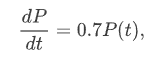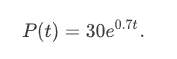# Validation

Course subject(s) 1. Introducing Mathematical Modelling

In the previous section you have seen that the solution of the differential equationwith the initial condition P(0)=30 is given byNow you will validate this solution. Or in other words: does this solution represent a realistic solution to our problem?